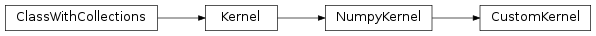# mvpa2.kernels.base.CustomKernel¶class `mvpa2.kernels.base.``CustomKernel`(kernelfunc=None, *args, **kwargs)

Custom Kernel defined by an arbitrary function

Examples

Basic linear kernel >>> k = CustomKernel(kernelfunc=lambda a,b: numpy.dot(a,b.T))

Attributes

 `descr` Description of the object if any

Methods

 `add_conversion`(typename, methodfull, methodraw) Adds methods to the Kernel class for new conversions `as_ls`(kernel) `as_np`() Converts this kernel to a Numpy-based representation `as_raw_ls`(kernel) `as_raw_np`() Directly return this kernel as a numpy array. `as_raw_sg`(kernel) Converts directly to a Shogun kernel `as_sg`(kernel) Converts this kernel to a Shogun-based representation `cleanup`() Wipe out internal representation `compute`(ds1[, ds2]) Generic computation of any kernel `computed`(\*args, \*\*kwargs) Compute kernel and return self `reset`()

Initialize CustomKernel with an arbitrary function.

Parameters: kernelfunc : function Any callable function which takes two numpy arrays and calculates a kernel function, treating the rows as samples and the columns as features. It is called from compute(d1, d2) -> func(d1,d2) and should return a numpy matrix K(i,j) which holds the kernel evaluated from d1 sample i and d2 sample j enable_ca : None or list of str Names of the conditional attributes which should be enabled in addition to the default ones disable_ca : None or list of str Names of the conditional attributes which should be disabled

Attributes

 `descr` Description of the object if any

Methods

 `add_conversion`(typename, methodfull, methodraw) Adds methods to the Kernel class for new conversions `as_ls`(kernel) `as_np`() Converts this kernel to a Numpy-based representation `as_raw_ls`(kernel) `as_raw_np`() Directly return this kernel as a numpy array. `as_raw_sg`(kernel) Converts directly to a Shogun kernel `as_sg`(kernel) Converts this kernel to a Shogun-based representation `cleanup`() Wipe out internal representation `compute`(ds1[, ds2]) Generic computation of any kernel `computed`(\*args, \*\*kwargs) Compute kernel and return self `reset`()